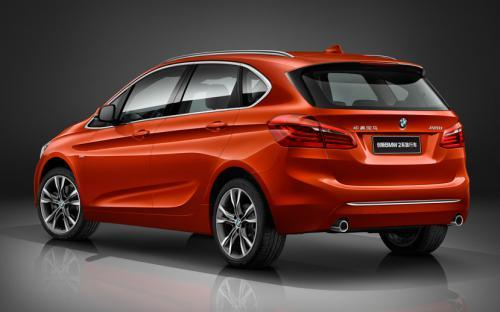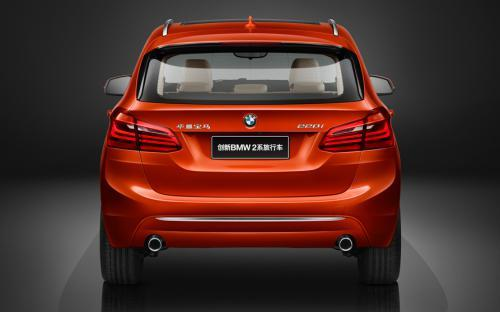### 华晨宝马 宝马2系旅行车11 种颜色可选2016款最低售价：23.69 万元起

4342(mm)1800(mm)1600(mm)##### 配置亮点：
• 胎压监测装置

• ISOFIX儿童座椅接口

• 车身稳定控制(ESC/ESP/DSC等)

• 电动天窗

• 定速巡航

• 后倒车雷达

• 真皮座椅

• GPS导航系统

• 氙气大灯

• 后视镜加热

• 提交
2016款 2016款 220i 豪华设计套装 (242张)
• 2016款 2016款 220i 豪华设计套装 (242张)
• 2016款 2016款 218i 运动设计套装 (132张)
• 华晨宝马 宝马2系旅行车 绕车实拍• 华晨宝马 宝马2系旅行车 在售车型

排量 车型 厂商指导价 本地最低报价 购车工具
1.5T
2016款 218i 时尚型 6挡手自一体
23.69万
23.69万

2016款 218i 领先型 6挡手自一体
24.99万
24.99万

26.99万
26.99万

2016款 220i 领先型 8挡手自一体
29.89万
29.89万

33.19万
33.19万

2.0T
2016款 218i 时尚型 6挡手自一体
23.69万
23.69万

2016款 218i 领先型 6挡手自一体
24.99万
24.99万

26.99万
26.99万

2016款 220i 领先型 8挡手自一体
29.89万
29.89万

33.19万
33.19万

华晨宝马 宝马2系旅行车 经销商

查看更多 >>

### 华晨宝马 宝马2系旅行车 动力加速

宝马2系旅行车 0-100公里加速时间分布在 7.5-9.4秒 属于 运动级

动力级别 加速时间 车型
民用级(1款)
9.4s
2016款 218i 时尚型2016款 218i 领先型2016款 218i 运动设计套装
运动级(1款)
7.5s
2016款 220i 领先型2016款 220i 豪华设计套装

华晨宝马 宝马2系旅行车 视频

华晨宝马 宝马2系旅行车 新闻资讯

# 新X1长轴距/M2等 宝马年内新车计划曝光

行业动态 超过7286次关注

日前，宝马集团大中华区新任总裁兼首席执行官康思远在采访中透露了2016年新车计划，今年，宝马将在中国推出10余款新车，其中包括4款新能源车，下面，让我们看一看年...

# 国产2系旅行车上市 售23.69-33.19万元

上市新车 超过7746次关注

3月21日华晨宝马正式宣布国产2系旅行车在国内上市，新车原汁原味的保持了进口车型的设计，提供两种动力共计五款车型供消费者选择，它们的售价区间为23.69-33.19万元...

# 3月21日上市 华晨宝马2系旅行车已到店

国产新车 超过7750次关注

华晨宝马2系旅行车将于3月21日正式上市。国产车型基本上与进口版并没有太大差别。基于UKL+前驱平台打造而来，目前在售的MINI同样也是UKL+平台下的产物，新车已经到...

# 增216i 国产宝马2系运动旅行车申报

国产新车 超过8308次关注

据官方获悉，我们的得到了一组国产宝马2系旅行车的谍照图，新车在外观方面基本延续了进口版车型的设计，搭载一台1.5T和2.0T发动机，匹配8速手自一体变速箱。

猜你喜欢

﻿
• 快速找车
• 选择品牌
• 选择品牌
• A  奥迪
• A  阿斯顿·马丁
• A  阿尔法·罗密欧
• B  宝沃
• B  布加迪
• B  巴博斯
• B  保时捷
• B  宾利
• B  奔驰
• B  宝马
• B  本田
• B  别克
• B  标致
• B  比亚迪
• B  宝骏
• B  北汽制造
• B  北汽新能源
• B  北汽幻速
• B  北汽威旺
• B  北京汽车
• B  奔腾
• B  北汽绅宝
• C  长安
• C  长安商用
• C  长城
• C  昌河
• D  大众
• D  道奇
• D  DS
• D  东南
• D  东风风神
• D  东风风行
• D  东风小康
• D  东风风度
• D  东风
• F  福特
• F  丰田
• F  菲亚特
• F  法拉利
• F  福田
• F  福迪
• F  福汽启腾
• G  观致
• G  广汽传祺
• G  广汽吉奥
• G  GMC
• H  红旗
• H  汉腾汽车
• H  哈弗
• H  哈飞
• H  海格
• H  海马
• H  华颂
• H  黄海
• H  华泰
• H  恒天
• J  吉利汽车
• J  捷豹
• J  Jeep
• J  江淮
• J  江铃
• J  金杯
• J  九龙
• J  金旅
• K  凯翼
• K  凯迪拉克
• K  克莱斯勒
• K  科尼塞克
• K  卡威
• K  开瑞
• L  路虎
• L  林肯
• L  劳斯莱斯
• L  兰博基尼
• L  雷克萨斯
• L  铃木
• L  雷诺
• L  理念
• L  力帆
• L  莲花汽车
• L  猎豹
• L  路特斯
• L  陆风
• M  马自达
• M  MG
• M  MINI
• M  玛莎拉蒂
• M  摩根
• M  迈凯轮
• N  纳智捷
• O  欧宝
• O  讴歌
• O  欧朗
• Q  奇瑞
• Q  起亚
• Q  启辰
• R  日产
• R  荣威
• R  瑞麒
• S  三菱
• S  斯威汽车
• S  萨博
• S  smart
• S  斯柯达
• S  斯巴鲁
• S  思铭
• S  双龙
• S  上汽大通
• S  双环
• T  特斯拉
• T  腾势
• W  沃尔沃
• W  五菱汽车
• W  五十铃
• W  威兹曼
• W  威麟
• X  现代
• X  雪佛兰
• X  雪铁龙
• X  西雅特
• Y  一汽
• Y  英菲尼迪
• Y  英致
• Y  依维柯
• Y  野马汽车
• Y  永源
• Z  众泰
• Z  中华
• Z  中兴
• Z  知豆
• 选择车系
• 选择车系
• 车型对比
• 选择品牌
• 选择品牌
• A  奥迪
• A  阿斯顿·马丁
• A  阿尔法·罗密欧
• B  宝沃
• B  布加迪
• B  巴博斯
• B  保时捷
• B  宾利
• B  奔驰
• B  宝马
• B  本田
• B  别克
• B  标致
• B  比亚迪
• B  宝骏
• B  北汽制造
• B  北汽新能源
• B  北汽幻速
• B  北汽威旺
• B  北京汽车
• B  奔腾
• B  北汽绅宝
• C  长安
• C  长安商用
• C  长城
• C  昌河
• D  大众
• D  道奇
• D  DS
• D  东南
• D  东风风神
• D  东风风行
• D  东风小康
• D  东风风度
• D  东风
• F  福特
• F  丰田
• F  菲亚特
• F  法拉利
• F  福田
• F  福迪
• F  福汽启腾
• G  观致
• G  广汽传祺
• G  广汽吉奥
• G  GMC
• H  红旗
• H  汉腾汽车
• H  哈弗
• H  哈飞
• H  海格
• H  海马
• H  华颂
• H  黄海
• H  华泰
• H  恒天
• J  吉利汽车
• J  捷豹
• J  Jeep
• J  江淮
• J  江铃
• J  金杯
• J  九龙
• J  金旅
• K  凯翼
• K  凯迪拉克
• K  克莱斯勒
• K  科尼塞克
• K  卡威
• K  开瑞
• L  路虎
• L  林肯
• L  劳斯莱斯
• L  兰博基尼
• L  雷克萨斯
• L  铃木
• L  雷诺
• L  理念
• L  力帆
• L  莲花汽车
• L  猎豹
• L  路特斯
• L  陆风
• M  马自达
• M  MG
• M  MINI
• M  玛莎拉蒂
• M  摩根
• M  迈凯轮
• N  纳智捷
• O  欧宝
• O  讴歌
• O  欧朗
• Q  奇瑞
• Q  起亚
• Q  启辰
• R  日产
• R  荣威
• R  瑞麒
• S  三菱
• S  斯威汽车
• S  萨博
• S  smart
• S  斯柯达
• S  斯巴鲁
• S  思铭
• S  双龙
• S  上汽大通
• S  双环
• T  特斯拉
• T  腾势
• W  沃尔沃
• W  五菱汽车
• W  五十铃
• W  威兹曼
• W  威麟
• X  现代
• X  雪佛兰
• X  雪铁龙
• X  西雅特
• Y  一汽
• Y  英菲尼迪
• Y  英致
• Y  依维柯
• Y  野马汽车
• Y  永源
• Z  众泰
• Z  中华
• Z  中兴
• Z  知豆
• 选择车系
• 选择车系
• 选择车型
• 选择车型
• 意见反馈